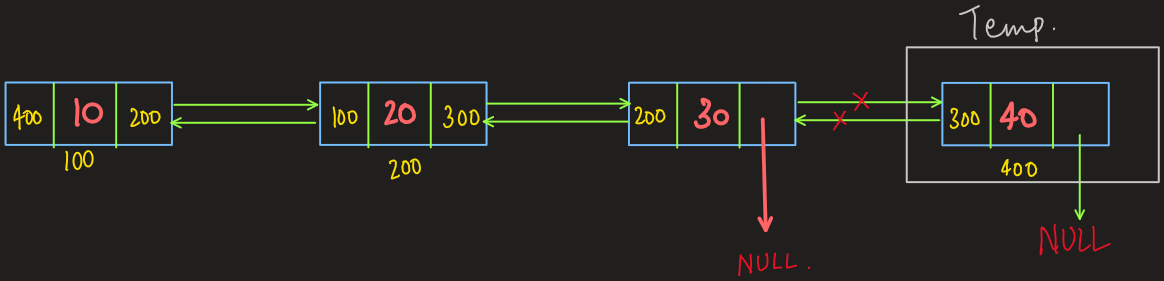# Delete Last Node of a Doubly Linked List

Problem Statement: Given a Doubly Linked List. Delete last of a Doubly Linked List.

Examples:

```Example 1:
Input: 10 20 30
Output: 10 20

Note: The above input represents a doubly linked list.

Explanation: Since the input represents a doubly linked list, “30” is the last node. After deletion, the linked list becomes “10 20”

Example 2:
Input: 10
Output: null

Explanation: Since “10” is the  only node of the doubly linked list which after deletion makes the linked list empty, hence we print “NULL”.

Example 3:
Input: NULL
Output: NULL

Explanation: Since the doubly linked list is empty, we print “NULL”.
```

DisclaimerDon’t jump directly to the solution, try it out yourself first.

## Solution:

### Intuition:

In this problem, we have to delete the last node of the doubly linked list. So let’s imagine a doubly linked list. When we think of the last node of a doubly linked list. It would have data, previous and next as its attributes. The next of the last node would point to NULL.

There are certain edge cases in this problem:

The first edge caseDLL is empty.

We return NULL if DLL is empty because we have no node to delete.

Second edge caseDLL consists of a single node.

We delete the single node since it is the only node in DLL and return NULL.

### Approach:

To delete the last node, we need to detach it from the linked list which can be done in a very simple way.

1. We traverse through the linked list to reach the last node.
2. We store our last node into a temporary node.
3. We change the next pointer of the new last node to point to NULL.
4. We delete the temporary node.

The following diagram may help in understanding the above approachCode:

## C++ Code

``````#include<iostream>

using namespace std;
struct node { // single node
int data;
node * next;
node * previous;
node(int d) { // constructor
data = d;
previous = NULL;
next = NULL;
}
};
node * delete_last(node * head) {
node * current = head;
while (current -> next != NULL) { // iterating to the last node
current = current -> next;
}
node * temp = current; // storing the last node
current -> previous -> next = NULL; // changing the next pointer of
neighboring node
delete temp; // deleting the last node
}
void print_dll(node * head) {
node * current = head;
while (current != NULL) {
cout << current -> data << " ";
current = current -> next;
}
cout<<endl;
}
int main() {
// creating nodes for the DLL
node * head = new node(10);
node * node1 = new node(20);
node * node2 = new node(30);

// 	constructing the DLL
head -> next = node1;
node1 -> previous = head;
node1 -> next = node2;
node2 -> previous = node1;
cout<<"Original Doubly Linked List"<<endl;
cout<<"After deleting the Last node"<<endl;
return 0;
}
``````

Output:

Original Doubly Linked List
10 20 30
After deleting the Last node
10 20

Time Complexity: O(size of the DLL)

Space Complexity: O(1)

## Java Code

``````import java.util.*;
import java.io.*;
import java.lang.*;

class node {
int data;
node previous;
node next;
node(int d) {
data = d;
previous = null;
next = null;
}
}

class DLL {

public static void main(String args[]) {
// creating nodes for the DLL
node head = new node(10);
node node1 = new node(20);
node node2 = new node(30);

// constructing the DLL
node1.next = node2;
node2.previous = node1;
System.out.println("Original Doubly Linked List");
System.out.println("After deleting the Last node");
print_DLL(head); // utility function

}

static node delete_last(node head) {
if (head == null) return null; //checks if the DLL is empty
if (head.next == null) { //checks if the DLL contains a single element
return null;
} else {
node current = head;
while (current.next != null) { // iterates through the DLL
current = current.next;
}
current.previous.next = null; //changes the next pointer of
the neighboring node
}
}

public static void print_DLL(node head) {
node current = head;
while (current != null) {
System.out.print(current.data + " ");
current = current.next;
}
System.out.println("");
}
}
``````

Output:

Original Doubly Linked List
10 20 30
After deleting the Last node
10 20

Time Complexity: O(size of the DLL)

Space Complexity: O(1)

Special thanks to Yash Mishra for contributing to this article on takeUforward. If you also wish to share your knowledge with the takeUforward fam, please check out this article